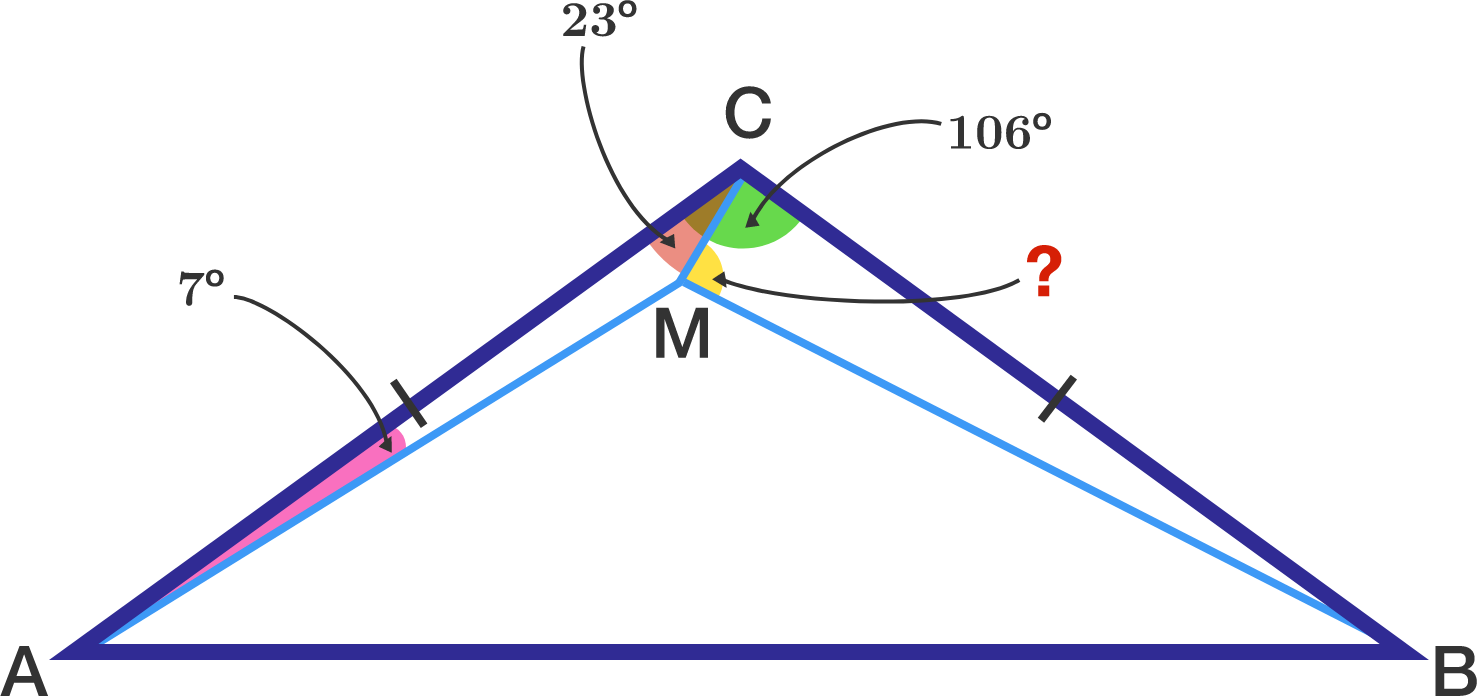# It's beautiful!

Geometry Level 3

Triangle $ABC$ is isosceles with $AC = BC$ and $\angle ACB = 106^\circ$ Point $M$ is inside the triangle so that $\angle MAC = 7^\circ$ and $\angle MCA = 23^\circ$. Find the measure of $\angle CMB$ in degrees.×

Problem Loading...

Note Loading...

Set Loading...